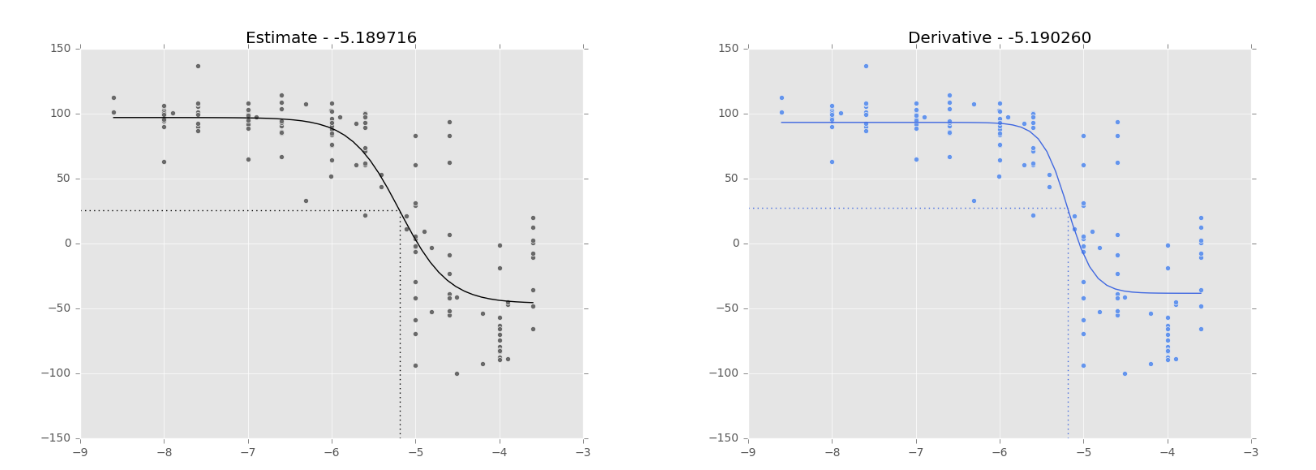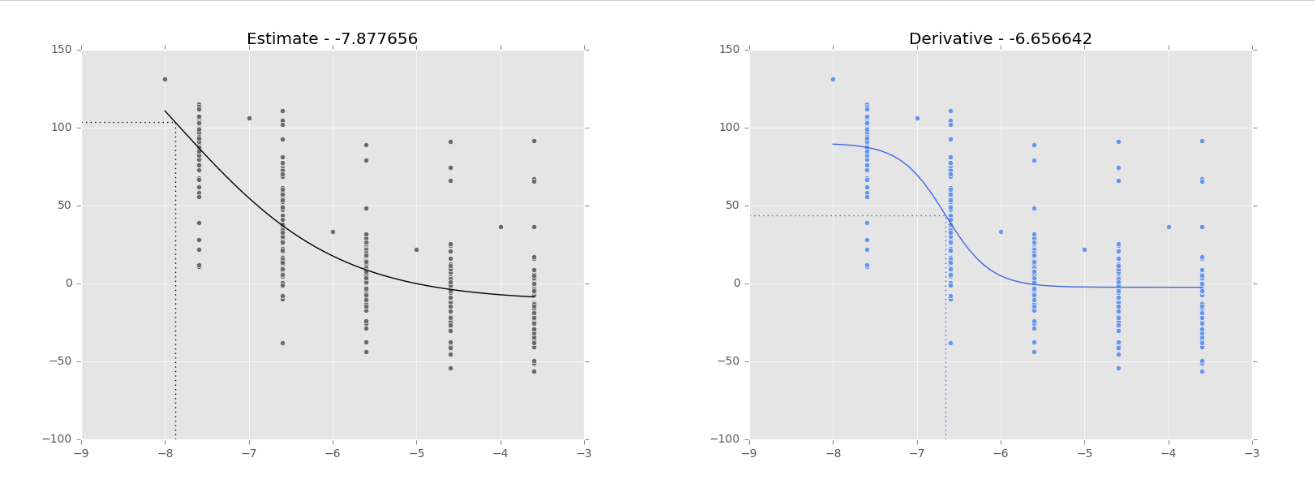Given a set of data points, we often want to see if there exists a satisfying relationship between them. Linear regressions can easily be visualized with Seaborn, a Python library that is meant for exploration and visualization rather than statistical analysis. As for logistic regressions, SciPy is a good tool when one does not have his or her own analysis script.

Let’s look at the optimize package
from scipy.optimize import curve_fit

Given n empirical datum pairs of independent and dependent variables (xi,yi), curve_fit is using nonlinear least square to fit any function f. The most basic call will look like
                       scipy.optimize.curve_fit(func, x, y) where x and y are arrays of data, and where func is callable. The latter is very interesting since we have the liberty to define any model function with m parameters to fit. Curve_fit is calling leastq (another method from scipy.optimize), which is a wrapper around MINPACK’s Levenberg-Marquardt algorithm.

By default, the method used for optimization is ‘lm’ (i.e. Levenberg-Marquardt), which is usually the most efficient method, except when m < n and when the problem is constrained. In these cases, we specify the method to use between ‘trf’ and ‘dogbox’ (Trust Region Reflective algorithm and dogleg algorithm, respectively)
                        scipy.optimize.curve_fit(func, x, y, method='trf')

When using other methods than ‘lm’, curve_fit will call least_square rather than leastq. That being said, the arguments of leastq and least_square can all be used by curve_fit, even if they do not appear in the documentation of this last optimization method. In other words, many functionalities can be found in the documentation of leastq and least_square.

Scipy.optimize.curve_fit(func, x, y) will return an array of the optimal estimated values for every parameter, and a matrix (2D array) of the estimated covariance of the parameters. Given these, we can easily plot our fitted curve.

The resulting curve might not be exactly what we would expect. Before jumping to any conclusion, let’s look at some arguments of curve_fit:

• f = func : we should always make sure that our model equation is well written; missing parentheses can create a whole new function!
• p0 =
[…] : by default, the initial guesses for the parameters are all set to 1. This guess could be far from the actual parameters, resulting in the algorithm to converge to false optimal values. We can initialize the parameters ourselves (note that our guesses could also be wrong).
• Dfun = dfunc : by default, the Jacobian of our function is estimated by MINPACK. We can define a callable similar to our func. When the data is ‘good’, the ‘estimate’ and the ‘derivative’ seem to behave similarly (Fig 1.). However, when the data is ‘bad’, the results are very different (Fig 2.).Figure 1. Logistic regression on NCI-60 data (180973_Leukemia_CCRF-CEM) to fit a dose-response curve. The dotted lines show the inflection point, as calculated by SciPy.optimize.curve_fit, which has its value in the title of the graph. Black curve: curve fitting based on Jacobian estimation (default). Blue curve: curve fitting based on a function to calculate the Jacobian (Dfun = dfunc). Similar curves when the data is ‘good’.Figure 2. Logistic regression on NCI-60 data (180973_Leukemia_CCRF-CEM) to fit a dose-response curve. The dotted lines show the inflection point, as calculated by SciPy.optimize.curve_fit, which has its value in the title of the graph. Black curve: curve fitting based on Jacobian estimation (default). Blue curve: curve fitting based on a function to calculate the Jacobian (Dfun = dfunc). Different curves when the data is ‘bad’.

SciPy is a good tool when it comes to logistic regressions. That being said, we should test different approaches before drawing any conclusion. For example, we could compare the least-square of ‘estimate’ and ‘derivative’ to see which one is better, or if their results are equivalent. We could also look at the percentile bootstrap confidence interval of the estimated parameters.